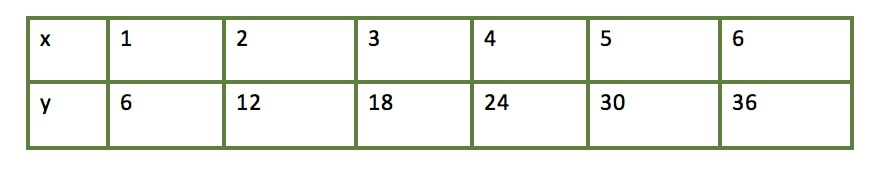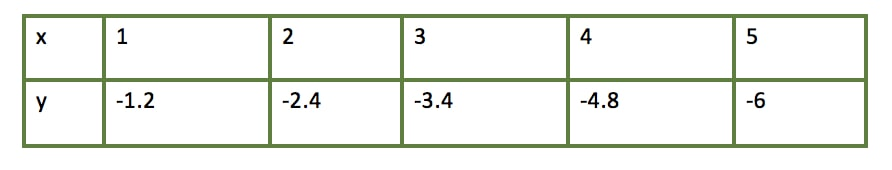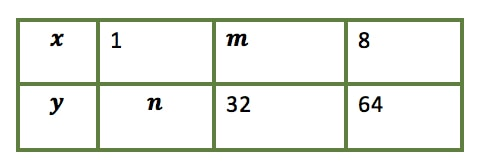# Direct variation#### Everything You Need in One Place

Homework problems? Exam preparation? Trying to grasp a concept or just brushing up the basics? Our extensive help & practice library have got you covered.#### Learn and Practice With Ease

Our proven video lessons ease you through problems quickly, and you get tonnes of friendly practice on questions that trip students up on tests and finals.#### Instant and Unlimited Help

Our personalized learning platform enables you to instantly find the exact walkthrough to your specific type of question. Activate unlimited help now!##### Examples
###### Lessons
1. Identifying direct variations by table of values
Do the following tables of values represent direct variation?1. Identifying direct variations
Which of the following represent direct variation?

A. $y$ = 2

B. $y$ = 3.1$x$

C. $y = - \frac{7x}{9}$

D. $xy$ = 191

E. $y$ = 8$x$ + 5
1. Variable $y$ varies directly with $x$. Determine $m$ and $n$1. If $a$ varies directly as $b$, and $b$ = 4 when $a$ = 16, find $a$ when $b = \frac{3}{2}$
1. If the volume of an object varies directly as the cube of its height, and the volume equals 40$ft^{3}$ when the height is 2$ft$, find the object's volume when its height equals $\frac{1}{3} ft$
###### Topic Notes
Direct Variation:
$y$ varies directly with $x$ with a constant variation of $k$

$y = kx$

where
$k \neq$ 0